# waynehu

## Professor, Department of Astronomy and Astrophysics University of Chicago

Group Contact CV SnapShots

### Physical Description

After recombination, without the pressure of the photons, the baryons simply fall into the Newtonian potential wells with the cold dark matter, an event usually referred to as the end of the Compton drag epoch. We claimed in §3.5 that above the horizon at matter-radiation equality the potentials are nearly constant. This follows from the dynamics: where pressure gradients are negligible, infall into some initial potential causes a potential flow of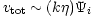[see Equation (19)] and causes density enhancements by continuity of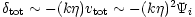. The Poisson equation says that the potential at this later time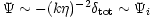so that this rate of growth is exactly right to keep the potential constant. Formally, this Newtonian argument only applies in general relativity for a particular choice of coordinates [Bardeen, 1980], but the rule of thumb is that if what is driving the expansion (including spatial curvature) can also cluster unimpeded by pressure, the gravitational potential will remain constant.

Because the potential is constant in the matter dominated epoch, the large-scale observations of COBE set the overall amplitude of the potential power spectrum today. Translated into density, this is the well-known COBE normalization. It is usually expressed in terms of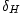, the matter density perturbation at the Hubble scale today. Since the observed temperature fluctuation is approximately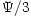,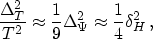(25)

where the second equality follows from the Poisson equation in a fully matter dominated universe with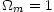. The observed COBE fluctuation of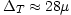K [Smoot et al, 1992] implies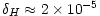. For corrections for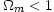where the potential decays because the dominant driver of the expansion cannot cluster, see [Bunn & White, 1997].

On scales below the horizon at matter-radiation equality, we have seen in §3.5 that pressure gradients from the acoustic oscillations themselves impede the clustering of the dominant component, i.e. the photons, and lead to decay in the potential. Dark matter density perturbations remain but grow only logarithmically from their value at horizon crossing, which (just as for large scales) is approximately the initial potential,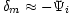. The potential for modes that have entered the horizon already will therefore be suppressed by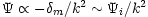at matter domination (neglecting the logarithmic growth) again according to the Poisson equation. The ratio of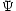at late times to its initial value is called the transfer function. On large scales, then, the transfer function is close to one, while it falls off as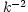on small scales. If the baryon fraction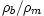is substantial, baryons alter the transfer function in two ways. First their inability to cluster below the sound horizon causes further decay in the potential between matter-radiation equality and the end of the Compton drag epoch. Secondly the acoustic oscillations in the baryonic velocity field kinematically cause acoustic wiggles in the transfer function [Hu & Sugiyama, 1996]. These wiggles in the matter power spectrum are related to the acoustic peaks in the CMB spectrum like twins separated at birth and are actively being pursued by the largest galaxy surveys [Percival et al, 2001]. For fitting formulae for the transfer function that include these effects see [Eisenstein & Hu, 1998].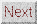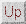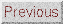Next: Cosmological Implications Up: Matter Power Spectrum Previous: Matter Power Spectrum
Wayne Hu 2001-10-15